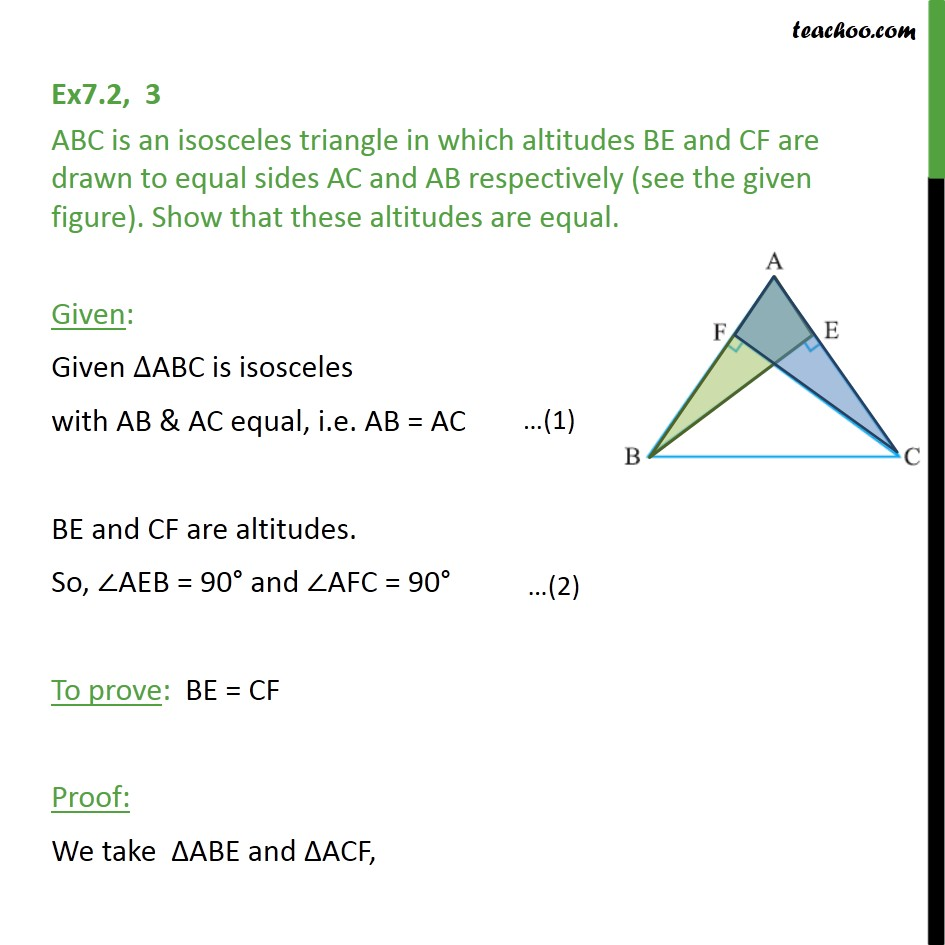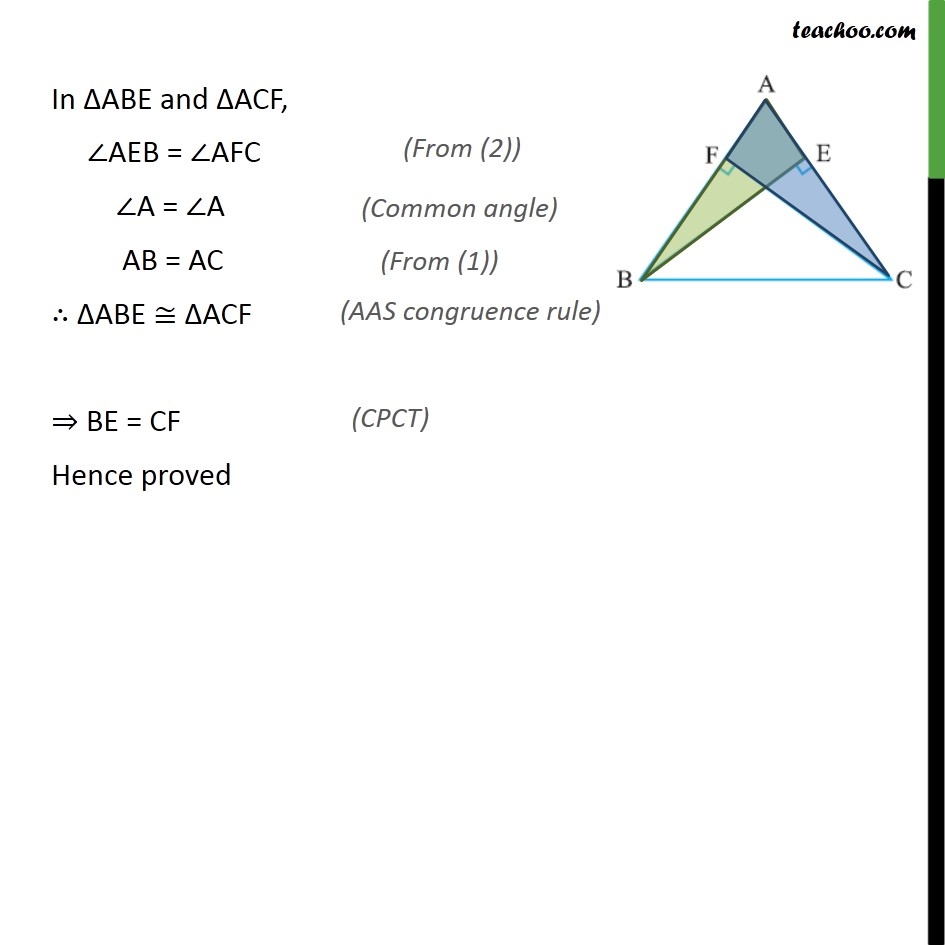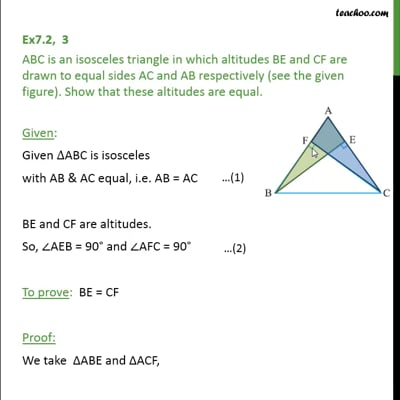Ex 7.2

Chapter 7 Class 9 Triangles
Serial order wiseThis video is only available for Teachoo black users

Maths Crash Course - Live lectures + all videos + Real time Doubt solving!

### Transcript

Ex7.2, 3 ABC is an isosceles triangle in which altitudes BE and CF are drawn to equal sides AC and AB respectively (see the given figure). Show that these altitudes are equal. Given: Given ABC is isosceles with AB & AC equal, i.e. AB = AC BE and CF are altitudes. So, AEB = 90 and AFC = 90 To prove: BE = CF Proof: We take ABE and ACF, In ABE and ACF, AEB = AFC A = A AB = AC ABE ACF BE = CF Hence proved